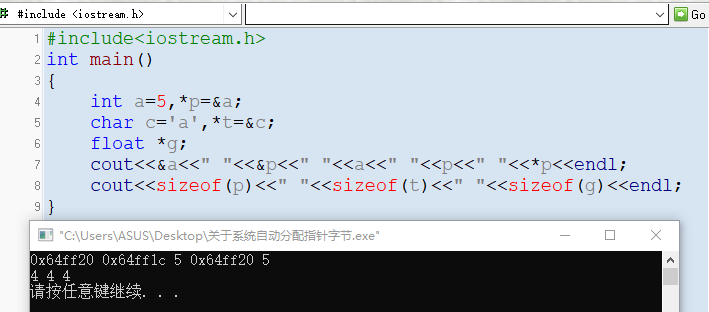# 关于系统自动给指针分配空间

#include<iostream.h>
int main()
{
int a=5,*p=&a;
char c=‘a’,*t=&c;
float *g;
cout<<&a<<" “<<&p<<” “<<a<<” “<<p<<” “<<*p<<endl; cout<<sizeof§<<” “<<sizeof(t)<<” "<<sizeof(g)<<endl;
}©️2019 CSDN 皮肤主题: 游动-白 设计师: 上身试试